Our Home Page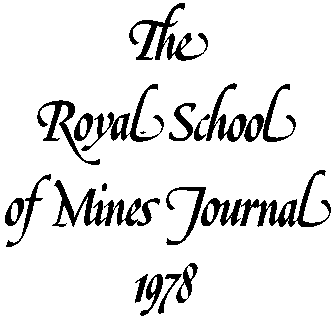Geostatistics by Isobel Clark

 Mrs. ISOBEL CLARK, M.Sc., F.S.S., F.I.M.M. Isobel Clark has been a Lecturer in the Mining section of the Mineral Resources Engineering Department for five years. Originally a statistician, she found life too boring in the Civil Service, and obtained her current post via a two year contract as Research Assistant in Statistics Applied to Mineral Resources. Her main field of interest is in the applications of statistical and geostatistical methods of ore reserve estimation in metalliferous mines. Mrs. Clark has been a Fellow of the Royal Statistical Society since 1970, and in recognition of her interdisciplinary status, the Institution of Mining and Metallurgy granted corporate Membership in August 1976.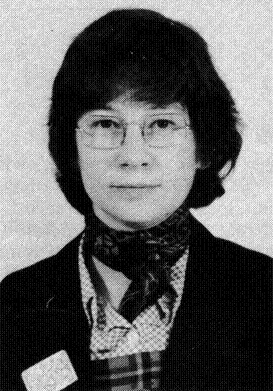Perhaps it would be best to start this article with an explanation of what I mean by "geostatistics". In the North American continent and some other parts of the world, the word has come to mean 'anything vaguely statistical applied to anything vaguely geological'. This is a definition which I would wholeheartedly support. However, over the last fifteen years or so a different meaning has been disseminating itself from the Centre de Morphologie Mathematique in Fontainebleau. The initiates of Georges Matheron's Theory of Regionalised Variables have perhaps a sound practical reason for wishing to shorten the description of their mentor's major work. Thus the title "Geostatistics" is now commonly used to denote the application of Matheron's theory in practice - and most frequently in Ore Reserve Estimation. It is my intention here to give a brief description of the main useful branches of Geostatistics, and to outline some of the ways in which it may ease the lot of the practicing reserves estimator.

The estimation of reserves has always been a necessary consideration in mine planning. What use an intricately planned and highly efficient installation which runs out of decent ore before the investment begins to pay off? But it is only recently (in mining world terms) that the yo-yoing metal prices and the decrease in easily found and highly payable deposits have pushed the reserves question into the forefront. Many consultancy firms and large mining companies now have sophisticated computer packages which will design them optimal open pits and/or underground installations. They can also produce complex economic analyses of these plans and test their sensitivity to all sorts of parameters. However, these companies are becoming more aware of just how delicately all this investment in computers and software is balanced on the accuracy (or otherwise) of their block-by-block estimation of the in-situ reserves. Many have attempted to remedy the situation by allowing the "user" to specify how, what, why or whether to use the company's chosen technique. This way any criticism of misuse or of arbitrary decisions can be shovelled off onto those who "know the deposit best". At the other end of the scale, we have the "small" mine, with one or two members of staff fitting in the reserves between other duties. Again, the reserves are produced by (hopefully) the one most fit to produce them, the one most familiar with the ore. Also, the methods used can be geared to the method of production, the scheduling, the local conditions, etc. All this is traditional (?) and properly acceptable - even if it does put we geostatisticians out of a job! The one thing these approaches do not do is to provide any quantitative measure of the confidence to be placed in the reserve estimates produced. Perhaps a qualitative assessment of the past efforts and/or successes of the practitioner will produce a confidence in his/her work. Considering the time span between the estimation of reserves and the production of the ore - and the usual impossibility of checking back on the original estimates - this could place severe constraints on the useful life of such a worker. A bad one may be found out swiftly (comparatively speaking), but how long does it take to prove a good one?

Most mine plans at the feasibility or at the production planning stages are produced on a block-by-block (open pit) or stope-by-stope basis. These local, mineable reserves can be amalgamated to produce global reserves and grade/tonnage curves. Sometimes, at an early stage, only the global figures are needed but I would like to confine my discussion to local reserve estimations. Consider the following situation: a block is to be estimated, and we have a sprinkling of samples in and around it. Such a setup is shown in Figure 1 below.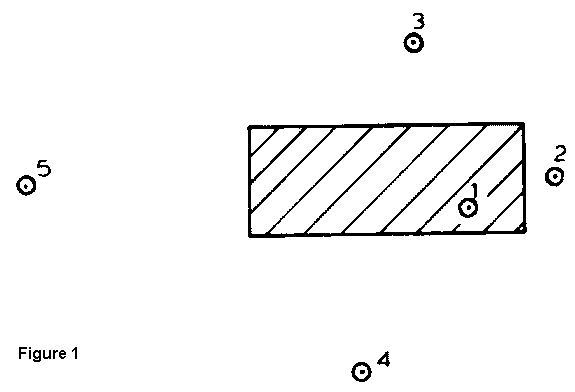It seems intuitively sound to expect that the grade of sample 1 will be rather similar (but not identical) to the average grade of the block. We could also expect that sample 2 would be fairly similar to the block, but less so than 1. Samples 3 and 4 are further away, but from the spread of the samples we might expect them to have some "influence" on the block value - or at least some relationship to it. Sample 5 is yet further away, and we must rely on knowledge of this deposit and/or the techniques being used to decide whether it should be included, and by how much to reduce its importance. In short, common sense tells us that samples close to the block should be highly related to it, and samples further away should be less so. It also seems sound that if we go too far from the block all relationship ceases. Samples further away than this distance have no influence on the block. Thus the notion of range of influence is introduced and accepted as sensible.

This notion of influence being in some way inversely related to the distance between two points is agreeable and easily assimilated. This does not necessarily mean that it is suspect. Some proponents argue the relative merits of distance, distance-squared, range of influence minus distance, and so on, but it all boils down to the same thing. We produce an estimator for the block average which is a weighted average of the sample values, with the weights being inversely related to the distance of the sample from the centre of the block. At some distance from this centre we stop considering any further samples. This raises two immediate problems. What happens if a sample sits exactly on the centre? What value does one divided by zero have? Should we allocate all the weight to the central sample, or still include other close ones? That is an easy one. Shift it one-quarter of the way to the corner. Why? Because that is what is done. Secondly, where do we stop taking samples. Leave that to the user! He knows what is relevant to his deposit, doesn't he? On the other hand, why should the same technique work equally well on porphyry coppers, nickel laterites, cassiterite veins, uranium deposits and so on and ad infinitum.

The approach described above involves some pretty fundamental assumptions when examined closely. The weighted average must be standardised so that we do not consistently over- or under-estimate the block values. This is usually coped with by setting the sum of the weights to one. This in itself carries one very basic assumption -- that there is no significant "trend" in values within the area under consideration. This means that there must be no change in the "expected" grade within a circle whose radius is our 'range of influence'. There may be rich areas and poor areas within the deposit -- in fact, we would look a bit silly doing all those sophisticated mine plans otherwise -- but we need to assume that these areas exist on a much larger scale than our block estimation procedure. In addition to this we assume that the relationships between samples and block are dictated only by geometrical position, and not by any local physical or statistical behaviour of the actual grade values. We also assume that these relationships are constant wherever the block (and samples) lie in the deposit, or indeed in any deposit. This can be modified slightly to allow for known geological differences in various parts of the deposit, but must still hold within these areas.

So far I have talked of the "relationship" between the samples and the block. If we think of "similarity" this is immediately linked with distance -- but inversely. If instead we think in terms of "difference in grade" this will be directly related to distance. The further away a sample gets, the more different it becomes from the block grade. When the sample gets beyond the range of influence, the difference reaches a constant level. This is speaking in ideal terms, of course. In reality, the differences will vary about the ideal, but on average should be about right. For many reasons, some of them practical, we prefer to consider the square of the difference in grades rather than the actual difference. We then only consider half of that. If we have enough samples in the right sort of pattern, we can calculate these squared differences, average them over the deposit, and of course half the answer. In this manner, it is possible to build up a graph of the "semi-variogram" versus the distance separating the samples. This semi-variogram will describe the difference in grade between any two samples a given distance apart. We can then assume that the difference between a sample and the centre of a block will follow the same sort of relationship. Thus we have a graph, constructed on this particular deposit, which tells us just how to weight our samples, because it tells us the difference between the samples and the block centre. A deposit suitable for weighting by inverse distance will give a straight line semivariogram, for instance.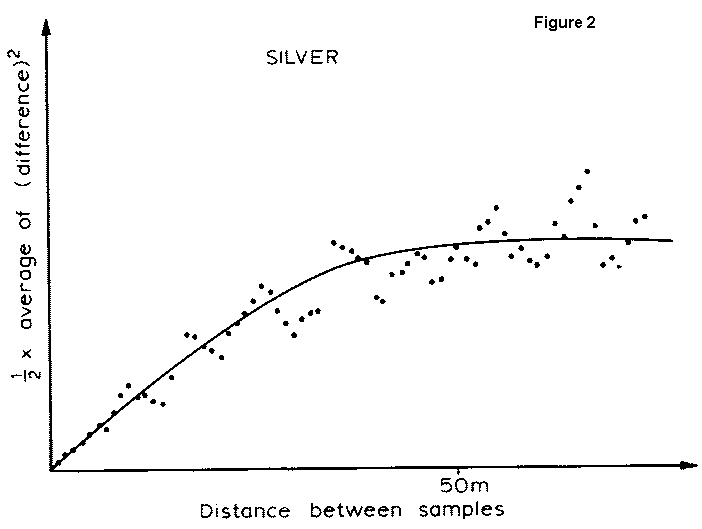Figure 2 shows such a graph calculated on channel samples taken along a 400 metre adit driven into a tabular, heavily disseminated, base metal sulphide deposit. The samples were taken at lm intervals, so that the calculation is child's play. The measurements used are per cent silver in each sample (by weight). The dots on the graph represent the average squared difference over all the samples divided by two. The smooth line is an idealised semivariogram, and would be the one actually used in the estimation procedure. This follows exactly the behaviour described above as desirable. Beyond a separation of 50m samples are independent. Below that distance we have a simple line to describe the exact (?) relationship between samples, and hopefully between a sample and the centre of a block.

The next graph shows another semi-variogram calculated on a nickel deposit. The "model" line in this case is more complex, but still reasonably simple. Note, however, that the line does not go through the origin of the graph. This implies that even at very small distances (less than one metre), there are still reasonably large differences in grade values. This implies that, no matter how closely we sample the deposit, there will always be a "random" component interfering with the estimates. This behaviour was first encountered in gold mines, and was explained by considering two points very close together - one inside a gold nugget and one outside. This would produce large differences even at such small distances. The effect is therefore called "nugget effect", even though it has since been encountered in all sorts of other deposits. Other suggestions are that the "unpredictable" component is actually sampling and/or analytical error. If you could take exactly the same sample twice, the actual measurement of grade might not be exactly the same. However, the jargon term nugget effect is still used in this case.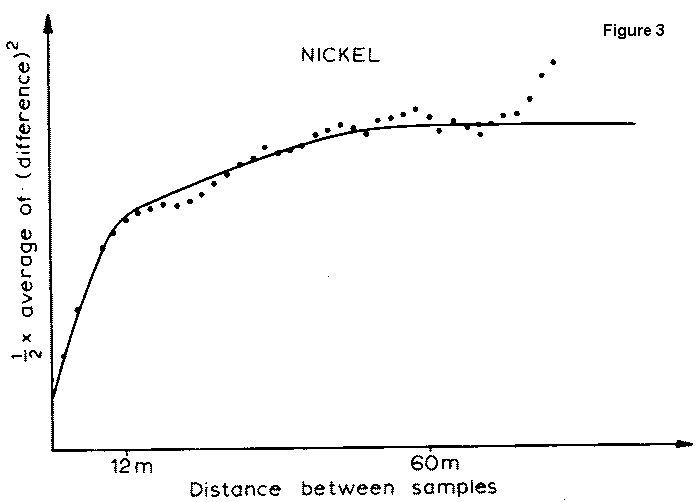Figure 4 shows a semi-variogram of the zinc values from another complex base metal sulphide deposit. To my eyes, this looks like pure 'nugget effect'. A smooth line drawn through that would be horizontal. It shows that no weighting technique is going to be of any use, since no spatial relationship exists between the samples. Definitely a case for a good deal more investigation.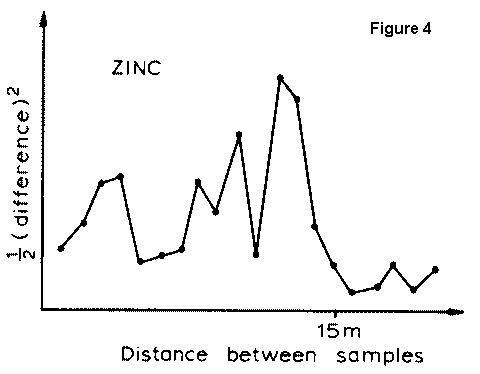To summarise then, a semi-variogram is useful in quantifying the 'difference in grade' between any two positions in a particular deposit. Each deposit yields its own semi-variogram, and thus the weighting of samples can be keyed to that deposit and its behaviour. Returning to figure 1, we need only measure the distance between each sample and the centre of the block; read off the value of the semivariogram at that distance; invert the values and standardise so that they sum to one. Now consider figure 5. The samples are in the same positions, and so is the centre of the block, but I have rotated the block through 90 degrees. If we repeat the previous procedure, we get the same weights as before. This is not very sensible. Sample 3 which was a good way outside the block now lies on its edge. Sample I which was inside is now outside. The relationship between the samples and the block average has obviously changed. What we need is not the semi-variogram between each sample and the centre point, but between the sample and the whole block.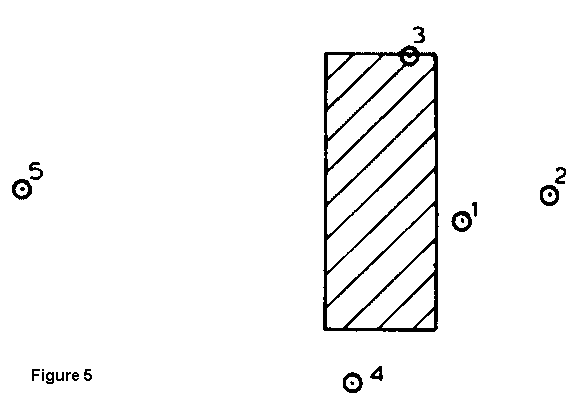Well, it so happens that having got our "model" semivariogram, it takes no great effort to produce semi-variograms for any given shape and/or size of sample and/or block. Even the final year miners can do it -- at least for the three hours of the exam! Figures, tables, charts etc. are available to ease the task, and the orientation, size and shape of the block (and the samples) can be incorporated automatically into the procedure. As a useful by-product, this removes the problem of central samples, since we can evaluate the actual relationship between a central point and the whole block.

There is still (at least) one more problem which may arise. Figure 6 shows the same setup as Figure 1, except that sample 3 is now on the same side of the block as sample 4. This raises two questions. Firstly, should the weighting remain the same, or should we adjust for the fact that two samples are very close together, and hence duplicating information.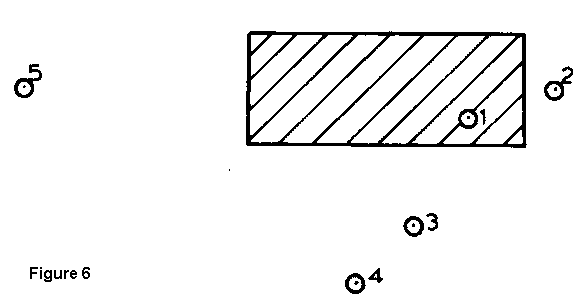I have seen this problem tackled by involving the cosine of the angle between samples (presumably subtended at the block centre) with the inverse distance weighting. Rather clumsy. The other question is, how much less reliable is the estimate of the block estimate in the latter case. The whole question of a quantitative measure of reliability is one which I have side-stepped so far. In classical statistics the usual measure of reliability is the 'standard error of the mean'. From this confidence limits may be produced. In our case we need the 'standard error of the weighted mean' for a set of samples which are not (by any stretch of the imagination) independent. In fact, the 'standard error' measures the difference between the estimator and the true value being estimated. To put it another way, we need some measure of the difference between the weighted average grade from the samples and the average grade of the block. This begins to sound suspiciously like a semi-variogram. In fact, if you plod through the mathematics, the 'standard error' is the square root of twice the semi-variogram between the samples (allowing for the different weights) and the block. It seems almost too neat and tidy.

To take the question of reliability to its logical conclusion, we would define the "best" estimator to be the most reliable. That is, it would have the lowest I standard error'. Since the standard error depends on the weights accorded to each sample, we merely need to find that set of weights which minimises the standard error - or the semi-variogram between the samples and the block. The mathematics produces a set of simultaneous equations whose solution yields the "best" weighting factors. On the right hand side of the equations are all the semi-variograms between each individual sample and the block. On the left hand side of the equations, intermingled with the unknown weights, are all the semi-variograms between each two samples. Not only the relationship between sample and block is considered, but also relationships amongst the samples. Figures 1, 5 and 6 would all produce different and optimal estimators for the block average. This procedure is called Kriging (kridging?, kreeking?, krigeage?, krigaggio?). Given the semi-variogram describing your deposit, it will automatically return the best estimator for any given position or block within the deposit. It will also tell you how 'good' that estimator is, by providing the standard error of the estimate as a by-product of solving the equations.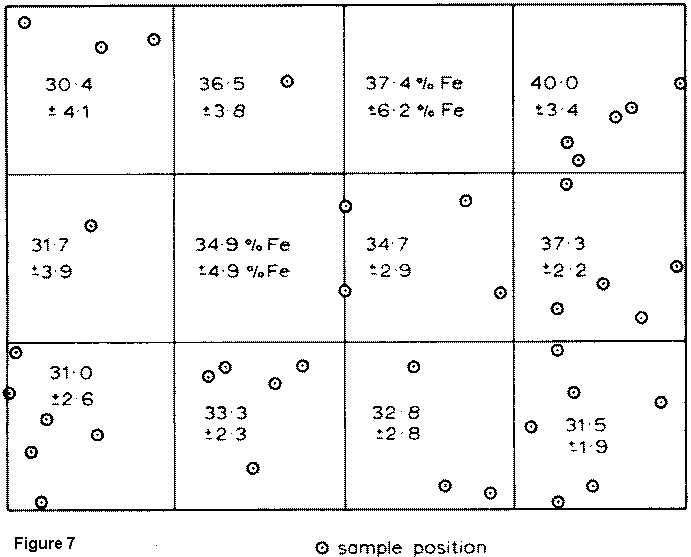Figure 7 shows a typical result of the kriging procedure used on one bench of a sedimentary iron ore deposit. Each block has an estimated mean grade, which should be optimal given the size and shape of the block and the positions of the sample values in and around it. The 'kriging standard error' has been used to produce a 95% confidence interval about each estimated value, and this is also shown in the diagram. In the block which contains no interior samples, for example, the estimated grade is 37.4% Fe, but we can only be 95% sure that the true value lies somewhere between 31.2% Fe and 43.6% Fe. At the other end of the scale, the lower right hand block (which contains six samples, and has several others close to it) gives a 95% confidence interval of between 29.6% Fe and 33.4% Fe.

Many arguments are used against the application of geostatistics in practical situations. It is too complicated and incomprehensible, they say. I should imagine some of my third year would agree with that - I hope not all of them. It doesn't work on my deposit, they say. Have you tried it? It is too expensive, they say. A friend of mine in the U.S. Geological Survey tells me that they replaced their inverse distance program by a kriging one, and it was cheaper. It was developed in a foreign country, they say. Well ........

Our Home Page